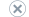# Aggregations and Aggregation Designs

Applies to:SQL Server Analysis ServicesAzure Analysis ServicesPower BI Premium

An AggregationDesign object defines a set of aggregation definitions that can be shared across multiple partitions.

An Aggregation object represents the summarization of measure group data at certain granularity of the dimensions.

A simple Aggregation object is composed of: basic information and dimensions. Basic information includes the name of the aggregation, the ID, annotations, and a description. The dimensions are a collection of AggregationDimension objects that contain the list of granularity attributes for the dimension.

Aggregations are precalculated summaries of data from leaf cells. Aggregations improve query response time by preparing the answers before the questions are asked. For example, when a data warehouse fact table contains hundreds of thousands of rows, a query requesting the weekly sales totals for a particular product line can take a long time to answer if all the rows in the fact table have to be scanned and summed at query time to compute the answer. However, the response can be almost immediate if the summarization data to answer this query has been precalculated. This precalculation of summary data occurs during processing and is the foundation for the rapid response times of OLAP technology.

Cubes are the way that OLAP technology organizes summary data into multidimensional structures. Dimensions and their hierarchies of attributes reflect the queries that can be asked of the cube. Aggregations are stored in the multidimensional structure in cells at coordinates specified by the dimensions. For example, the question "What were the sales of product X in 1998 for the Northwest region?" involves three dimensions (Product, Time, and Geography) and one measure (Sales). The value of the cell in the cube at the specified coordinates (product X, 1998, Northwest) is the answer, a single numeric value.

Other questions may return multiple values. For example, "How much were the sales of hardware products by quarter by region for 1998?" Such queries return sets of cells from the coordinates that satisfy the specified conditions. The number of cells returned by the query depends on the number of items in the Hardware level of the Product dimension, the four quarters in 1998, and the number of regions in the Geography dimension. If all summary data has been precalculated into aggregations, the response time of the query will depend only on the time that is required to extract the specified cells. No calculation or reading of data from the fact table is required.

Although precalculation of all possible aggregations in a cube might provide the fastest possible response time for all queries, SQL Server Analysis Services can easily calculate some aggregrated values from other precalculated aggregations. Additionally, calculating all possible aggregations requires significant processing time and storage. Therefore, there is a tradeoff between storage requirements and the percentage of possible aggregations that are precalculated. If no aggregations are precalculated (0%), the amount of required processing time and storage space for a cube is minimized, but query response time may be slow because the data required to answer each query must be retrieved from the leaf cells and then aggregated at query time to answer each query. For example, returning a single number that answers the question asked earlier ("What were the sales of product X in 1998 for the Northwest region") might require reading thousands of rows of data, extracting the value of the column used to provide the Sales measure from each row, and then calculating the sum. Moreover, the length of time required to retrieve that data will very depending on the storage mode chosen for the data-MOLAP, HOLAP, or ROLAP.

## Designing Aggregations

Microsoft SQL Server SQL Server Analysis Services incorporates a sophisticated algorithm to select aggregations for precalculation so that other aggregations can be quickly computed from the precalculated values. For example, if the aggregations are precalculated for the Month level of a Time hierarchy, the calculation for a Quarter level requires only the summarization of three numbers, which can be quickly computed on demand. This technique saves processing time and reduces storage requirements, with minimal effect on query response time.

The Aggregation Design Wizard provides options for you to specify storage and percentage constraints on the algorithm to achieve a satisfactory tradeoff between query response time and storage requirements. However, the Aggregation Design Wizard's algorithm assumes that all possible queries are equally likely. The Usage-Based Optimization Wizard lets you adjust the aggregation design for a measure group by analyzing the queries that have been submitted by client applications. By using the wizard to tune a cube's aggregation you can increase responsiveness to frequent queries and decrease responsiveness to infrequent queries without significantly affecting the storage needed for the cube.

Aggregations are designed by using the wizards but are not actually calculated until the partition for which the aggregations are designed is processed. After the aggregation has been created, if the structure of a cube ever changes, or if data is added to or changed in a cube's source tables, it is usually necessary to review the cube's aggregations and process the cube again.